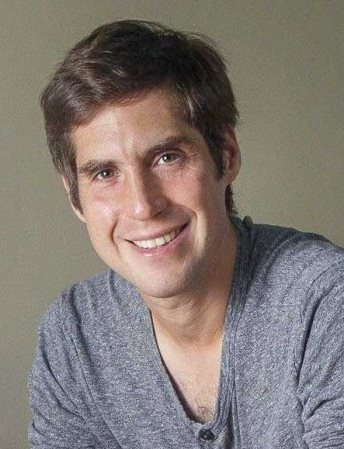distinguished lecture series presents

### Harvard University

###### Research Area

Algebraic Geometry, Topology, Homotopy Theory

###### Visit

Tuesday, October 16, 2018 to Thursday, October 18, 2018

MS 6627

# Website

Let L be a positive definite lattice. There are only finitely many positive definite lattices L’ which are isomorphic to L modulo N for every N > 0: in fact, there is a formula for the number of such lattices, called the Smith-Minkowski-Siegel mass formula. In the first lecture, I’ll review the Siegel mass formula and explain a reformulation (due to Tamagawa and Weil) in terms of the volumes of certain adelic groups. This reformulation led Weil to conjecture a generalization of the mass formula, which applies to any (simply connected) semisimple algebraic group G over any global field K. In the second lecture, I’ll discuss the meaning of this conjecture in the case where K is a function field (that is, a finite extension of F_p(x), for some prime number p), and explain how it can be reformulated as a statement about the cohomology of certain moduli spaces. In the third lecture, I’ll discuss recent joint work with Dennis Gaitsgory, applying ideas from algebraic topology to compute the relevant cohomology groups and thereby obtain a proof of Weil’s conjecture in the function field case.
###### abstracts
The Siegel Mass Formula and Weil’s Conjecture
Weil’s Conjecture for Function Fields
Weil’s Conjecture via Factorization Homology
###### Lecture 3
2300 Murphy Hall - Box 951438 - Los Angeles, CA 90095-1438 © 2018